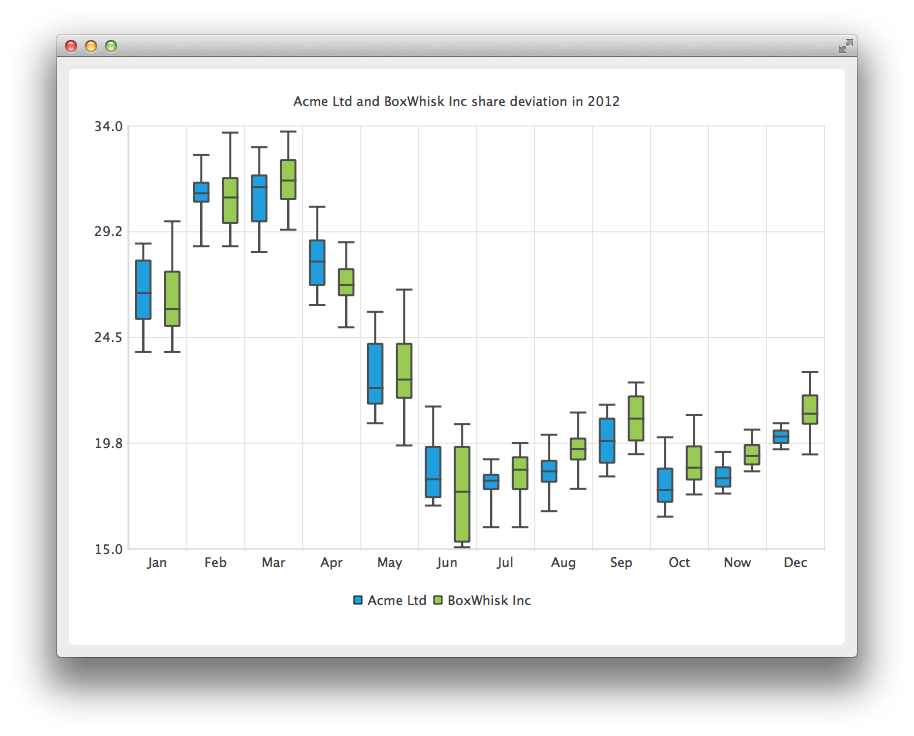# Box and Whiskers Example

The example shows how to create a box-and-whiskers chart.

The example also shows how to read the non-continuous data from a file, arrange it and find medians needed for box-and-whiskers plotting.#### Running the Example

To run the example from Qt Creator, open the Welcome mode and select the example from Examples. For more information, visit Building and Running an Example.

#### Creating Box-and-Whiskers Charts

To show the share deviation of two companies we start by creating two QBoxPlotSeries to handle monthly data.

```QBoxPlotSeries *acmeSeries = new QBoxPlotSeries();
acmeSeries->setName("Acme Ltd");

QBoxPlotSeries *boxWhiskSeries = new QBoxPlotSeries();
boxWhiskSeries->setName("BoxWhisk Inc");```

QFile class is used to open a text file where the non-continuous data is kept. The BoxDataReader is an auxiliary class for reading the text file and finding the extreme and median values from the data. The BoxDataReader is explained in more detail later. The method readBox reads the values and sets them to the QBoxSet item which the method returns for the caller. The returned QBoxSet item is added to the series.

```QFile acmeData(":acme");
return 1;

if (set)
acmeSeries->append(set);
}```

In this section a second file is opened for reading the data for the second company.

```QFile boxwhiskData(":boxwhisk");
return 1;

if (set)
boxWhiskSeries->append(set);
}```

In this code snippet a new QChart instance is created and previously created series are added to it. The title is also defined and animation is set to be SeriesAnimation.

```QChart *chart = new QChart();
chart->setTitle("Acme Ltd and BoxWhisk Inc share deviation in 2012");
chart->setAnimationOptions(QChart::SeriesAnimations);```

Here we ask the chart to create default axes for our presentation. We also set the range for the vertical axis by querying the pointer for the axis from the chart, and then setting the min and max for that axis.

```chart->createDefaultAxes();
chart->axes(Qt::Vertical).first()->setMin(15.0);
chart->axes(Qt::Horizontal).first()->setMax(34.0);```

In this section we set the legends to be visible and place them at the bottom of the chart.

```chart->legend()->setVisible(true);
chart->legend()->setAlignment(Qt::AlignBottom);```

Finally, we add the chart onto a view. We also turn on the antialiasing for the chartView.

```QChartView *chartView = new QChartView(chart);
chartView->setRenderHint(QPainter::Antialiasing);```

The chart is ready to be shown. We set the chart to be the central widget of the window. We also set the size for the chart window and show it.

```QMainWindow window;
window.setCentralWidget(chartView);
window.resize(800, 600);
window.show();```

Here the method readBox is explained in detail. Firstly, a line is read from the file and lines starting with # are rejected since they are considered as comment lines.

```QString line = readLine();
if (line.startsWith("#"))
return 0;```

In this file the data is arranged as number, space, number, or space. On this snippet the line is split into single number strings which are stored on QStringList.

`QStringList strList = line.split(QLatin1Char(' '), Qt::SkipEmptyParts);`

The sortedList will hold the numbers in continuous order and in this code segment we show how to do it. First the sortedList is cleared and numbers are read from the strList and stored into sortedList in double format. The qSort method arranges the sortedList into continuous order starting from the smallest.

```sortedList.clear();
for (int i = 1; i < strList.count(); i++)
sortedList.append(strList.at(i).toDouble());

std::sort(sortedList.begin(), sortedList.end());```

Below you will find a code sample showing how to select extremes and medians from the continuous data. Firstly a new QBoxSet is created. Lower and upper extremes are simple to select; they are just first and last items on the sortedList. For medians we use a helper method findMedian which is explained later. For the median from the upper half we need to adjust the begin number if the amount of the numbers is even or uneven. The end number for lower half comes naturally from int rounding.

```QBoxSet *box = new QBoxSet(strList.first());
box->setValue(QBoxSet::LowerExtreme, sortedList.first());
box->setValue(QBoxSet::UpperExtreme, sortedList.last());
box->setValue(QBoxSet::Median, findMedian(0, count));
box->setValue(QBoxSet::LowerQuartile, findMedian(0, count / 2));
box->setValue(QBoxSet::UpperQuartile, findMedian(count / 2 + (count % 2), count));```

Below you will find the code sample for the method findMedian. If the amount of numbers is uneven we select the number from the middle. For even amount numbers we take two numbers from the middle and calculate the mean value.

```int count = end - begin;
if (count % 2) {
return sortedList.at(count / 2 + begin);
} else {
qreal right = sortedList.at(count / 2 + begin);
qreal left = sortedList.at(count / 2 - 1 + begin);
return (right + left) / 2.0;
}```

Example project @ code.qt.io